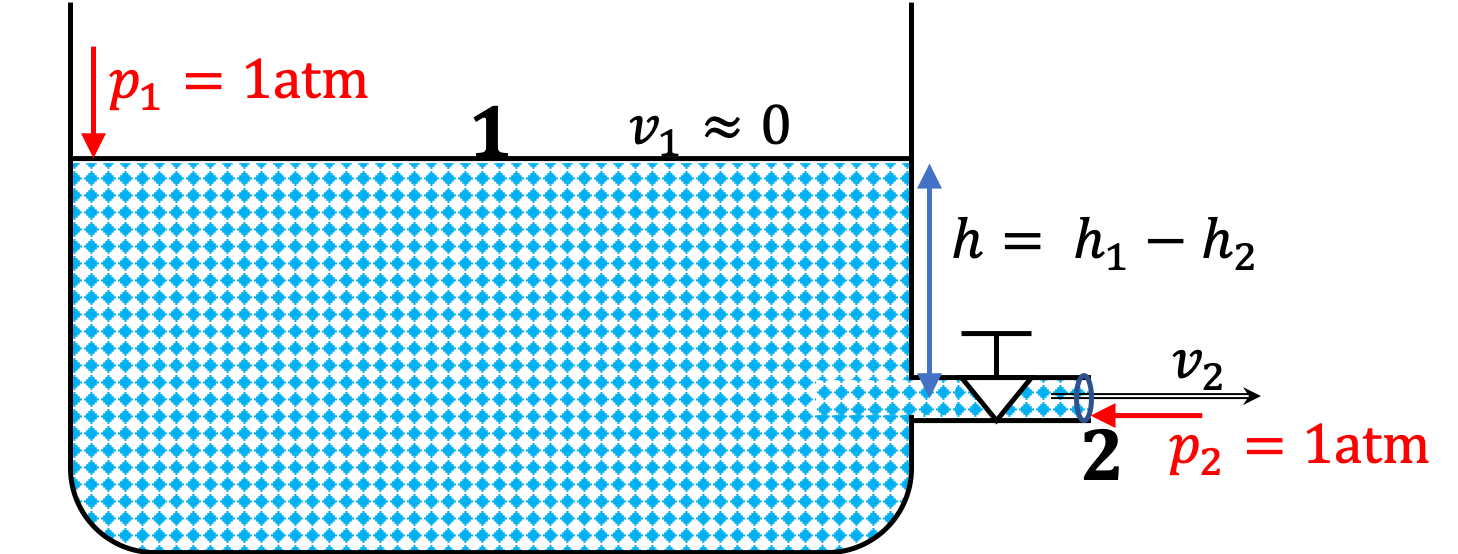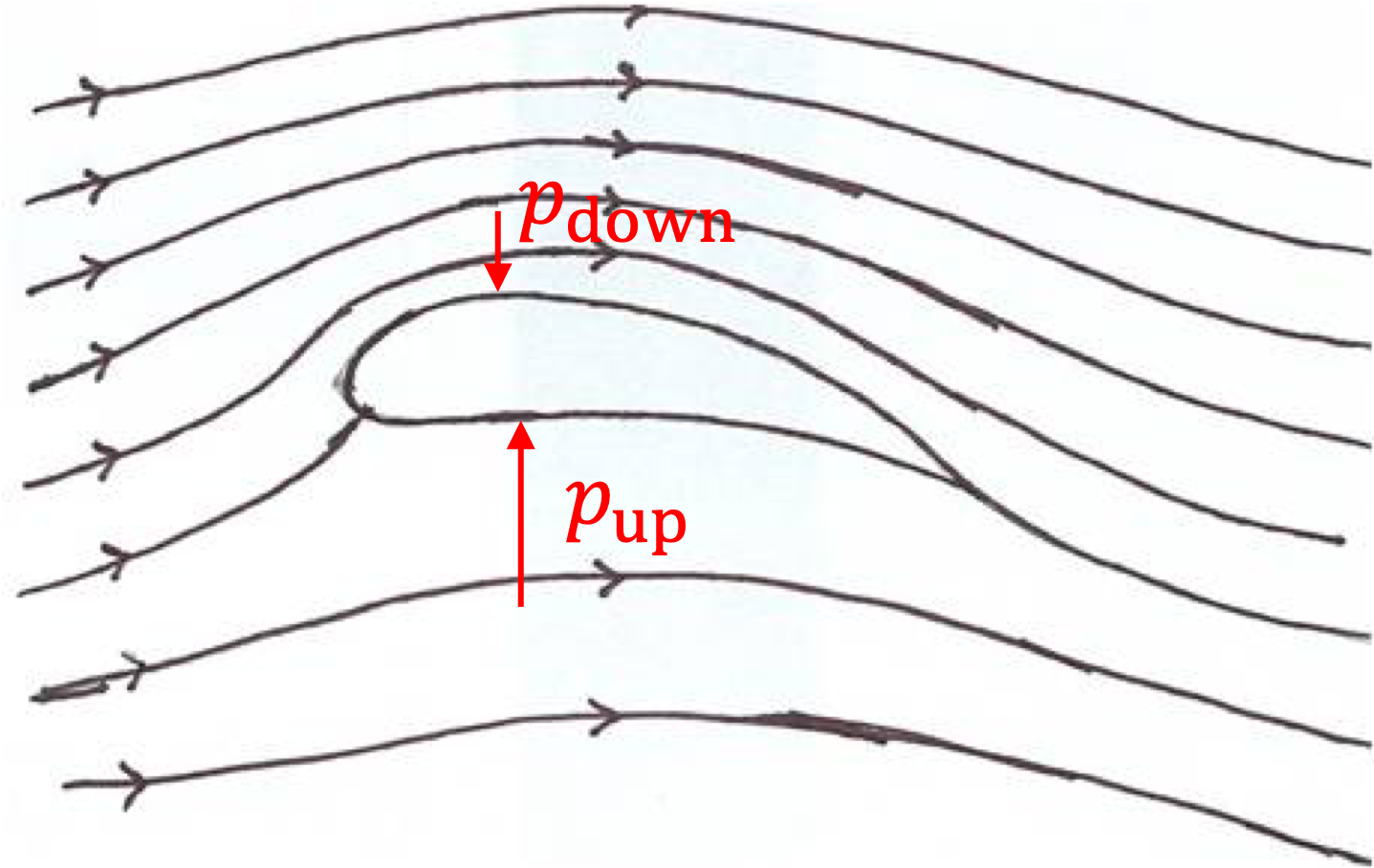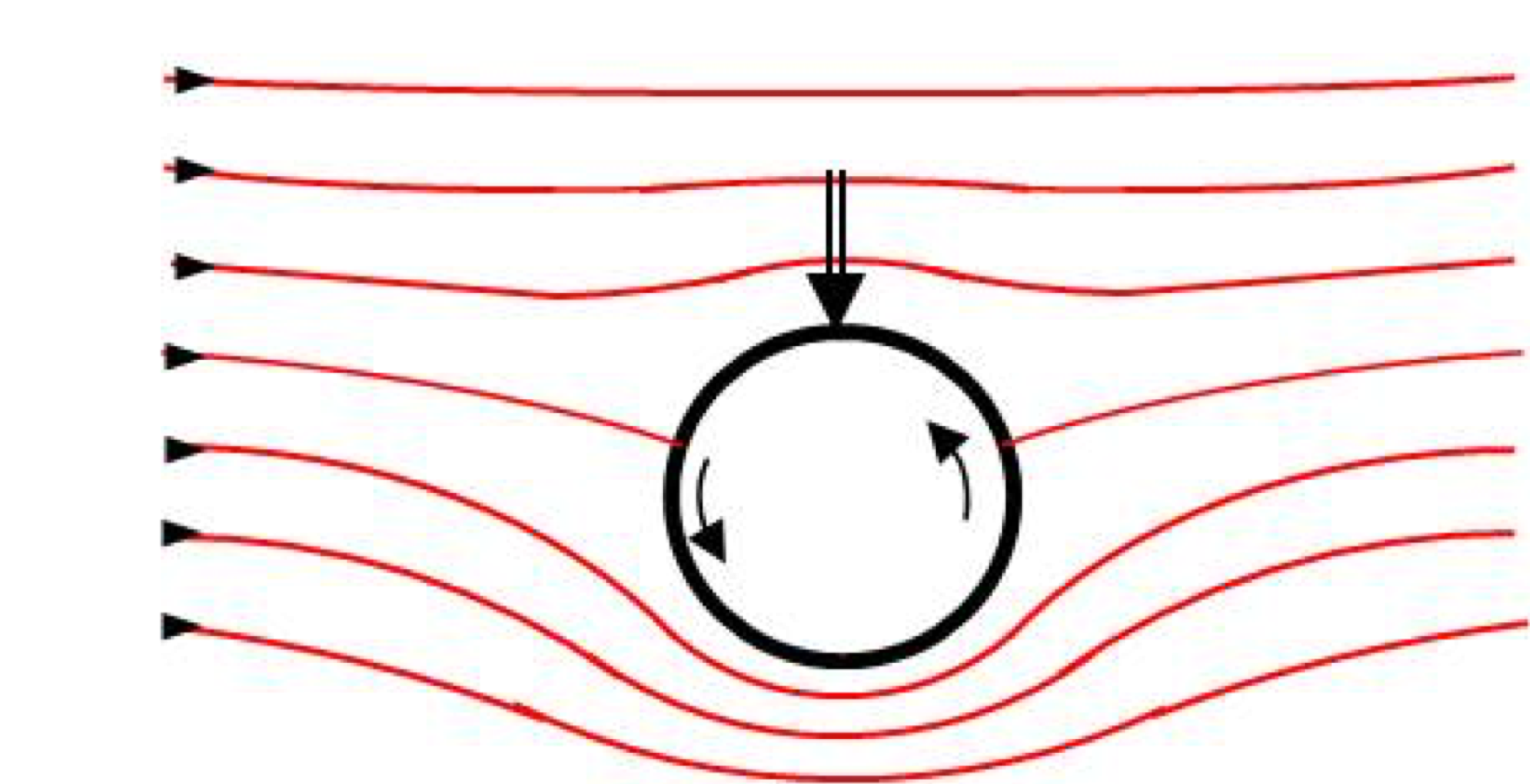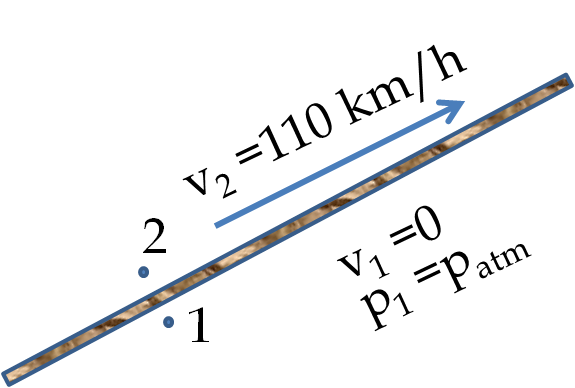## Section18.3Applications of Bernoulli's Equation

### Subsection18.3.1Bernoulli for Static Fluid or Same Speed at Two Ends

Bernoulli's equation includes static behavior as we can see immediately by setting $v_1 = v_2 = 0$ in the equation.

\begin{equation} p_1 + \rho g h_1 = p_2 + \rho g h_2. \ \ \text{(Static)}\tag{18.3.1} \end{equation}

If the area of pipe at the entrance and exit points are same, the speed of flow will be same, canceling the kinetic energy terms. This results in the same equation.

\begin{equation} p_1 + \rho g h_1 = p_2 + \rho g h_2. \ \ \text{(Same Pipe Size at Ends)}\tag{18.3.2} \end{equation}

This says that work by the pressure difference between two points in a fluid at rest of having same criss-sections, provides the difference in the potential energy.

\begin{equation} p_1 -p_1 = \rho g \left( h_2 - h_1 \right). \tag{18.3.3} \end{equation}

### Subsection18.3.2Same Pressure at Two ends

This tells us that energy entering at 1 must equal the energy leaving at 2.

\begin{equation} \dfrac{1}{2}\rho v_1^2 + + \rho g h_1 = \dfrac{1}{2}\rho v_2^2 + + \rho g h_2.\label{eq-torricelli-eqn-general}\tag{18.3.4} \end{equation}

Suppose we have a wide tank full of water with a narrow opening on the side. Let point 1 be the water level and 2 the exit at the narrow opening as indicated in Figure 18.3.1. The difference in area of cross-sections is so great here, i.e., $A_1 \gt\gt A_2\text{,}$ means $v_1 \approx 0\text{.}$Figure 18.3.1. Note that the water system is pushed by atmospheric pressure at 1 and also exiting at 2 against the same atmospheric pressure.

Note that at the faucet, the pressure $p_2 = 1\text {atm}\text{.}$ Its not $p_2 \ne 1\text {atm} + \rho g h\text{,}$ since we want the pressure against which the flow pushes, not the pressure inside the fluid before it comes out of the faucet.

With $v_2=0\text{,}$ $h = h_1 - h_2\text{,}$ and $p_1 = p_2\text{,}$ Eq. (18.3.4), ends up giving us a simple formula for the speed with which water will be exiting at the narrow opening.

\begin{equation*} v_1 = \sqrt{2gh}, \end{equation*}

which may remind you of the formula of the speed of a ball dropped by a height $h\text{,}$ which is not surprising since both invove conservation of energy during a fall by a height $h\text{.}$ This formula is sometimes referred to as the Torricelli's law.

### Subsection18.3.3Bernoulli for no Change in Height

For horizontally flowing case, $h_1=h_2\text{,}$ Bernoulli's equation simplifies.

\begin{equation} p_1 + \frac{1}{2}\rho v_1^2 = p_2 + \frac{1}{2}\rho v_2^2.\tag{18.3.5} \end{equation}

An important interpretation of this equation is that in a fluid, pressure is lower where the speed is higher and vice versa. This conclusion is quite “contrary to the common sense”. It would seem that pressure should be greater where the fluid is flowing at a higher speed than where it is flowing at a lower speed, but Bernoulli's equation tells us that just the opposite is true.

Following examples show applications to airplane lift and baseball spin. Similarly, in atmospheric physics, we find that the wind speed is higher where the pressure is lower, and in circulatory system, blood flows fastest where the pressure is the lowest.

To make use of this aspect of the flow of fluids, airplane wings are constructed so that air will flow with a greater speed above the wings than below the wings. This flow pattern gives rise to a lower pressure at the top of the wing than at the bottom.

Therefore, the force pushing on the wing from below would be larger than the force pushing down on the wing from the above. This difference in the force is called the lift force and is responsible for flying.

Air flows faster above the wing of an airplane than below the wing aided by the design of the foil. Therefore, pressure above the wing is less than below the wing. The product of the area of the wing and the difference in pressure is the lift force on the wing.Downward force on spinning curve baseball arises due to increased speed of the air flow at the bottom compared to the speed above the ball when the ball is spinning backwards.An air plane of mass 1000 kg has air of density $1.2\ \text{kg/m}^3$ flowing above the wing of area $15\ \text{m}^2$ at a speed of $60\text{ m/s}$ over the top surface and $50\text{ m/s}$ past the bottom surface.

(a) Find the lift force on the airplane wing.

(b) How does the lift force compare with the weight of the airplane?

Hint

(a) Use Bernoulli's equation, then use $A\Delta P$ to get the force. (b) Take difference to compare.

(a) $9,900\ \text{N} \text{,}$ (b) The lift force is $100$ N more than the weight.

Solution 1 (a)

Since the height between the top and bottom of the wing is negligible for the present purpose, we can find the pressure difference between the top and bottom of the wing by using simplified Bernoulli's equation.

\begin{align*} \amp p_1 + \frac{1}{2}\rho v_1^2 = p_2 + \frac{1}{2}\rho v_2^2\\ \amp p_{\text{bottom}} - p_{\text{top}} = \frac{1}{2}\rho_{\text{air}}\left( v_{\text{top}}^2 - v_{\text{bottom}}^2 \right) = 660\ \text{Pa}. \end{align*}

The magnitude of the lift force $F_L$ is the pressure difference times area of the wing.

\begin{equation*} F_L = 660\ \text{Pa}\times 15\ \text{m}^2 = 9,900\ \text{N}. \end{equation*}
Solution 2 (b)

(b) The weight of the plane is $W= 1000\ \text{kg}\times9.81\ \text{m/s}^2 =9800 \text{N}\text{.}$ Hence, in the present case, the lift force is $100$ N more than the weight.

The Venturi meter is a device for measuring the flow speed of gases and liquids of a known density $\rho\text{.}$ The fluid at some unknown speed $v_1$ enters at the inlet, as shown in Figure 18.3.6, passes through a constriction called the throat where a manometer is attached which contains a denser liquid of density $\rho_0\text{.}$ The areas of cross-section at the inlet and constriction are $A_1$ and $A_2$ respectively. The difference in levels $h$ is related to speed $v_1 \text{.}$ Find this relation.

Hint

Treat flow of fluid $\rho$ by Bernoulli equation and $\rho_0$ by static fluid techniques.

$\sqrt{\frac{2(x-1)gh}{\left( 1-y^2 \right)}}\text{.}$

Solution

Let $p_1$ be the pressure at the inlet and $p_2$ at the constricted point shown in the figure. Applying Bernoulli's equation to the flowing fluid of density $\rho$ at the inlet and outlet at the same height we obtain the following equation.

\begin{equation} p_1 + \frac{1}{2}\rho v_1^2 = p_2 + \frac{1}{2}\rho v_2^2.\label{eq-venturi-1}\tag{18.3.6} \end{equation}

Another relation between the pressures at the inlet and outlet is obtained by applying the static pressure condition on the fluid of density $\rho_0$ in the tube. Let $H$ be the height of flowing fluid above A. Then, we have $H-h$ the height of flowing fluid in the right side of the U tube. Equating the fluid pressure at A with that at B we get

\begin{equation} p_1 \rho g H = p_2+ \rho g (H-h) + \rho_0 g h.\label{eq-venturi-2}\tag{18.3.7} \end{equation}

Subtracting Eq. (18.3.7) from Eq. (18.3.6) we find

\begin{equation} \left( \rho_0-\rho \right) gh = \frac{1}{2}\rho v_2^2- \frac{1}{2}\rho v_1^2. \label{eq-venturi-3}\tag{18.3.8} \end{equation}

The equation of continuity for flowing gas between the inlet and outlet gives us the other relation we need to find $v_1\text{.}$

\begin{equation} A_1v_1 = A_2 v_2.\label{eq-venturi-4}\tag{18.3.9} \end{equation}

Eliminating $v_2$ from Eqs. (18.3.8) and (18.3.9), we find the following result for the speed of gas at the inlet.

\begin{equation} v_1 = A_1\sqrt{\frac{2(\rho_0-\rho)gh}{\rho\left( A_1^2-A_2^2 \right)}}. \label{}\label{eq-venturi-eqn}\tag{18.3.10} \end{equation}

Therefore, we can write $v_1$ in a more compact way

\begin{equation} v_1 = \alpha\,\sqrt{ h },\tag{18.3.11} \end{equation}

where $\alpha$ is a constant. Recording the difference in the two arms of the U-tube for the static fluid of density $\rho_0$ gives us $h$ which lets us determine the flow speed of the flowing fluid of density $\rho$ at the inlet by Eq. (18.3.10).

The formula in Eq \ref{eq:venturi-eqn} can be simplified by introducing the following ratios.

\begin{align*} \amp x \equiv \frac{\rho_0}{\rho},\\ \amp y \equiv \frac{A_2}{A_1}. \end{align*}

With these changes Eq (18.3.10) becomes

\begin{equation} v_1 = \sqrt{\frac{2(x-1)gh}{\left( 1-y^2 \right)}}, \tag{18.3.12} \end{equation}

which has the added advantage that we do not need to worry about the particular system of units for the densities and the areas because they cancel out in the ratios.

On a windy day wind blows on top of a roof at a speed of $110\text{ km/hr}\text{.}$ Under the roof air is quiet. If the density of air is $1.3\text{ kg/m}^3\text{,}$ what is the lift force per unit area of the roof.

Hint

Apply Bernoulli's principle across the roof.

$607\text{ N/m}^2.$

Solution

We apply Bernoulli's principle between two points as shown in the figure below. We can do this, even though, the flow is not the flow of a fluid in the same tube, since air on the two sides of the roof can flow around the roof into each other freely.The values of the parameters of interest are

\begin{align*} \amp h_2 -h_1 \approx 0 \\ \amp v_1 = 0\ \ \textrm{(just when starts to flow)} \\ \amp p_1 = p_{\textrm{atm}} = 1.013\times 10^{5}\ \textrm{Pa} \\ \amp v_2 = 110\ \textrm{km/h} = 30.6\ \textrm{m/s} \end{align*}

Therefore, we get

\begin{equation*} p_2 + \frac{1}{2}\rho v_2^2 = p_1. \end{equation*}

Putting in the numbers this gives

\begin{equation*} p_2 = p_1 - 607\ \textrm{Pa}. \end{equation*}

The magnitude of the lift force $F_L$ will be equal to the product of the the difference of pressure and the surface area $A\text{.}$ The lift force per unit area of the roof will have the magnitude,

\begin{equation*} \frac{F_L}{A} = |\Delta p| = 607\ \textrm{Pa}, \end{equation*}

and the direction would be from the inside to the outside.

A venturi flow meter shown in Figure 18.3.9 is used to measure the speed of water in a pipe of diameter $5\text{ cm}\text{.}$ The Venturi meter uses an oil with density $1.8\text{ g/cc}$ and at the consgtriction, the diameter is $1.5\text{ cm}\text{.}$ The difference in the height in the U-tube of the meter is $2\text{ cm}\text{.}$

Find the speed of flow of water in the $5\text{-cm}$ pipe.

Hint

Study Venturi meter in Example 18.3.5 first.

$58.7\text{ cm/s}$

Solution

There are two fluids in the venturi system, the manometer fluid of density $\rho_0$ and the flowing fluid of density $\rho\text{.}$ After utilizing the Bernoulli's equation between $A_1$ and $A_2$ and using static fluid formulas for the fluid $\rho_0$ it has been in the text that

\begin{align*} v_1 \amp = A_1\sqrt{\frac{2(\rho_0 - \rho) g h}{\rho(A_1^2 - A_2^2)}}\\ \amp = \sqrt{\frac{2(x - 1) g h}{(1 - y^2)}}, \end{align*}

where $x = \rho_0/\rho$ and $y = A_2/A_1 = d_2/d_1$ with $d_1$ and $d_2$ the diameters. In this exercise we have

\begin{align*} \amp \textrm{Diameter,}\ d_1 = 5\ \textrm{cm},\\ \amp \textrm{Diameter,}\ d_2 = 1.5\ \textrm{cm},\\ \amp y = 1.5/5 = 0.3. \end{align*}
\begin{align*} \amp \rho_0 = 1.8 \ \textrm{g/cc} = 1800 \ \textrm{kg/m}^3, \\ \amp \rho = 1.0 \ \textrm{g/cc} = 1000 \ \textrm{kg/m}^3\ \ (\textrm{water}), \\ \amp x = 1.8. \end{align*}
\begin{equation*} h = 2\ \textrm{cm}. \end{equation*}

Putting these values in the formula I got $v_1 = 58.7\text{ cm/s}\text{.}$